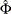### Printed Output

For each MODEL statement, the printed output from PROC PANEL includes the following:

• a model description, which gives the estimation method used, the model statement label if specified, the number of cross sections and the number of observations in each cross section, and the order of moving average error process for the DASILVA option. For fixed-effects model analysis, an F test for the absence of fixed effects is produced, and for random-effects model analysis, a Hausman test is used for the appropriateness of the random-effects specification.

• the estimates of the underlying error structure parameters

• the regression parameter estimates and analysis. For each regressor, this includes the name of the regressor, the degrees of freedom, the parameter estimate, the standard error of the estimate, a t statistic for testing whether the estimate is significantly different from 0, and the significance probability of the t statistic.

Optionally, PROC PANEL prints the following:

• the covariance and correlation of the resulting regression parameter estimates for each model and assumed error structure

• thematrix that is the estimated contemporaneous covariance matrix for the PARKS option